# RBSE Solutions For Class 12 Physics Chapter 4: Electrical Capacitance | Textbook Important Questions & Answers

The answers are provided for all the questions of Chapter 4 Physics of RBSE Class 12. Students can go through these questions to understand the concepts better and score well in the board examination and entrance examinations for various professional courses. These solutions are provided by the team of experts to provide the best and accurate solutions to the questions. Do check BYJU’S RBSE Class 12 solutions page to get the answers for textbook questions of all subjects.

## Multiple Choice Questions

Q1: If the plates of a charged capacitor are connected with a conducting wire, then

1. The potential will be infinite
2. Charge will be infinite
3. Charge will become double the initial value
4. Capacitor will be discharged

Q2: The ratio of the radius of two spherical conductors is 1:2, then the ratio of their capacitance will be

1. 4 : 1
2. 1 : 4
3. 1 : 2
4. 2 : 1

Q3: Eight mercury drops of equal radius and equal charge combine to form a big drop. The capacitance of the big drop in comparison each small drop will be

1. 2 times
2. 8 times
3. 4 times
4. 16 times

Q4: If the stored energy is Q on charging by charge Q. The stored energy on doubling the charge will be

1. 2 W
2. 4 W
3. 8 W
4. ½ W

Q5: Two spheres of 3 μF and 5 μF are charged by the potential of 300 V and 500 V respectively. The common potential will be

1. 400 V
2. 375 V
3. 425 V
4. 350 V

Q1: If the area of one plate of a parallel plate capacitor is halved then can the device be called a capacitor?

Answer: The device cannot be called a capacitor since both the plates are of unequal area.

Q2: What will be maximum and minimum capacitance obtainable with three capacitors each of 6μF capacitance?

(i) For maximum capacitance, the capacitors have to be connected in parallel

Cmax = C1+C2+C3

Cmax = 6μF +6μF +6μF =18μF

(ii) For minimum capacitance, the capacitors should be connected in series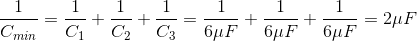Q3: Mention the factors affecting capacitance of a conductor

Answer: The capacitance of the capacitor depends on

(i) Area of cross-section

(ii) Medium surrounding the conductor

Q4: On assuming earth as a spherical conductor what will be its capacitance?

Answer: The capacitance of a spherical capacitor is given by

C = 4πє0R

R = 6400km

Є0 =8.85 x 10-12

Substituting the values we get C = 711μF

Q5: What is the electric field between the plates of a charged parallel plate capacitor with surface charge density σ?

Answer: The electric field is given by the value E=σ/Є0

Q6: If n capacitors of equal capacitance C are connected in series what will be the equivalent capacitance.

Answer: The resultant capacitance is given by the equation

Cresultant =C/n

Where C is the capacitance of the capacitor and n the number of capacitors

Q7: Write the expression for energy density for the electric field between plates of a parallel plate capacitor.

The expression for energy density is given by U/ V or U=(½)Є0E2

Q8: Write the unit of energy density

Answer: The unit of energy density is given by Joule/m3

Q9: Two capacitors of capacitance C1 and C2 are given equal charge write the ratio of electrostatic potential energy stored in them.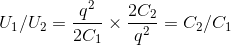Q10: Mention a conductor which can be given a nearly infinite amount of charge.

Answer: Earth can be given infinite charges. It has a very large capacitance

Q11: In which form the energy of the charged capacitor is stored?

Answer: Energy in a capacitor is stored as electric energy between the plates of capacitors

Q12: What is the net electric charge on a charged capacitor?

The net electric charge on a charged capacitor is zero

Q13: What is the basic use of the capacitor?

Answer: The capacitors are used to store electric energy and electric charge

Q14: If you have given capacitors of low capacitance. How will you get more capacitance from these capacitors?

Answer: If these capacitors are connected in parallel maximum capacitance can be got

Q15: The distance between the plates of a parallel plate capacitor is d. If a metallic plate of thickness d/2 is placed between the plates, what will be the effect on the capacitance?

Answer: For the metallic plate capacitance is given by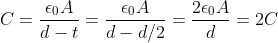Hence, capacitance is doubled if the metallic plate is placed between them

Q16: How much work is done to charge the capacitor of capacitance 24 μF when the potential difference between the plates is 500 V?

The work done is given by W = Vq

We know Charge q =CV

Therefore W=CV2

W=24 x 10-6x 500 X 500

W = 6 J

Q17: What is the equivalent capacitance when the capacitors of 2 μF are connected in series combination?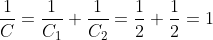Q18: What is the effect on capacitance on the parallel plate capacitor when the capacitor is immersed in oil? The dielectric constant of oil is 2.

The capacitance of any medium is given by Cm

Cm = KC0, where C0 is the capacitance of free space

The dielectric constant K=2

Therefore, the capacitance will be doubled

Q19: A circular parallel plate capacitor has radius r. Air is filled between the plates. If the capacitance of the capacitor is equal to that of the sphere of radius R, then what is the distance between the plates?

The capacitance of the parallel plate capacitor is

C=Є0A/d = Є0πr2/d

Where A=πr2 is the area of the plate,d is the separation between plates.

and radius of the plate is r

The capacitance of the spherical capacitor is given by

C=4πЄ0R

where R is the radius of the spherical capacitor.

According to the question

Capacitance of parallel plate = Capacitance of spherical surface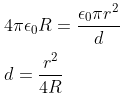Q1: Explain terms conductor and insulator with examples

(a)Conductors: Conductors are substances through which electric current flows easily. Conductors have free electrons which drift through the conductor. Silver, Copper, Iron are examples of good conductors

(b)Insulators: The substance through which electric current does not flow easily are called insulators. Examples of insulators are rubber, plastic, glass, etc.

Q2: Distinguish between polar and non-polar dielectrics

1. Polar dielectric: In this type of materials the centre of negative charge distribution and positive charge distribution does not coincide. The separation between centres causes these molecules to have a permanent dipole moment. Example of a polar molecule is water. When there is no electric field acting on the material the dipole are randomly oriented due to their random thermal motion. The net dipole moment will be zero. When the electric field is applied to this material the dipole will align with the field. Therefore, a dipole moment is developed in the material.
1. Non-Polar dielectrics: In this type of materials the centre of negative and positive charges coincide. They do not have a permanent dipole. An example of non-polar molecule is carbon dioxide. When an external electric field is applied negative charge distribution is shifted opposite to the electric field and positive charge in the direction of the electric field.

Q3: Obtain the formula for stored energy in a charged capacitor.

The capacitor is a device to store electrical energy.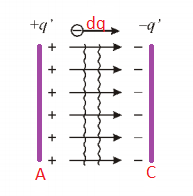Let us consider at a time t, a charge dq is transferred from a plate A to plate B.The potential difference (V) between the capacitor of capacitance C is given by

V=q/C

The work done in transferring the charge dq from one plate to another is given by

dW = Vdq

The work done is stored in the form of potential energy. The total work done in charging the capacitor from zero to Q is given by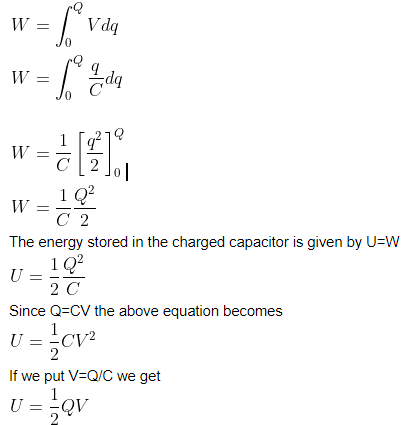Thus we get the energy stored in the capacitor

Q4: What will be the effect on the potential difference across the plates of a capacitor if its plates are brought nearer keeping the charge constant? Explain

The potential difference between the capacitors is inversely proportional to the capacitance

V∝

The capacitance is given by

C=

If the distance between the plates (d) decreases then the capacitance increases. Since capacitance and potential difference are inversely proportional, the potential difference decreases.

Q5: A parallel plate capacitor with air as the medium is charged to a potential difference V using a source (battery). Without disconnecting the battery air medium is replaced by a dielectric medium of dielectric constant εr. Explain with reasons the change observed in the following

(i) potential difference

(ii) the electric field between the plates

(iii) capacitance

(iv) charge

(v) energy

(i) This potential remains constant. When the battery is connected the potential difference will be equal to the potential of the battery.

(ii) The electric field is given by the equation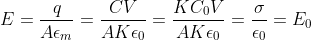The Electric field remains the same

(iii) The capacitance will be K times C0, where C0 is the capacitance of the air or vacuum

(iv) There will be an increase in charge since q=CV

(v) The energy stored in the capacitor is directly proportional to the capacitance

Hence, the energy stored also increases K times

Q6: A parallel plate capacitor with air as the medium is charged to a potential difference V using a source (battery). The battery is disconnected and the air medium is replaced by a dielectric medium of dielectric constant εr . Explain with reasons the change observed in the following

(i) potential difference

(ii) the electric field between the plates

(iii) capacitance

(iv) charge

(v) energy

After removing the battery

(i) The potential difference (V) decreases because the capacitance(C) increases since V∝

(ii) The Electric field decreases as the potential difference decreases since E=V/d

(iii) The capacitance will be equal to dielectric constant times

(iv) The charge will remain unchanged due to the conservation of charges

(v) The energy will decrease by the factor k

Q7: Three capacitors of capacitance C each are connected first in series and then in parallel. Calculate the ratio of equivalent capacitance in these two situations.

(i) In parallel

The capacitance is given by the sum of all the capacitance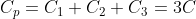(ii) If the capacitors are connected in series the capacitance is given by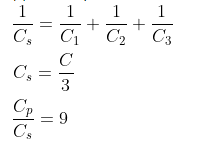Q8: n capacitors of capacitance C each, when connected in series, yields an equivalent capacitance Cs and when connected in parallel, yields an equivalent capacitance Cp.

Find the ratio of Cp and Cv.

(i)Cp=C1+C2+C3—-Cn=nC

(ii)The capacitance in series Cs=C/n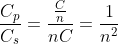Q9: What will happen to the capacitance of a spherical conductor if the charge on it is increased by three times? Give reasons

The capacitance of the spherical conductor is given by

C=4πє0R

The capacitance of the capacitor does not depend on the charge of the spherical conductor.

Q10: On filling the space between a 2μF air capacitor by mica its capacitance becomes 5μF. Calculate the dielectric constant of mica.

Answer: The capacitance of the medium is given by

Cm=KC0

Therefore K=Cm/C0

K=5/2

K= 2.5 μF

## Essay Type Question

Q1: Determine the formula for energy density between the plates of a parallel plate capacitor.

Let us consider a parallel plate capacitor of area A, and a charge Q is given to the plates. Then, the electric field between the plates

E=σ/Є0 = Q/Є0A

The charge density σ = Q/A

Therefore, Q = є0EA

But C=є0A/d

Where d is the distance between the plates

The energy of charged capacitor,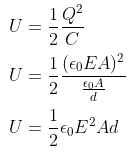Ad is the volume between the plates

Therefore,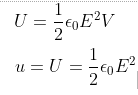The energy density is given the energy per unit volume

For a parallel plate capacitor, E and U do not vary with position in the region between capacitor plates

Q2: What is a spherical capacitor? Obtain the relation for the capacitance of a spherical capacitor.

Answer: Let us consider a spherical conductor of radius R placed in free space.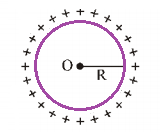A charge Q is given to the surface and the electric potential V at its surface is given by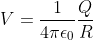The capacitance C is given by C=Q/V

C=4πЄ0R ——————————(1)

From the above equation, we understand capacitance is directly proportional to radius R

If the spherical conductor is kept in a medium of dielectric constant Єr then the capacitance will be given by

Cm =4πЄ0ЄrR ————————-(2)

From equation 1 and 2 we know

Cm =CЄr

Cm/C =Єr

Therefore the dielectric constant of the medium is equal to the capacitance of the conductor in the medium and the capacitance in free space.